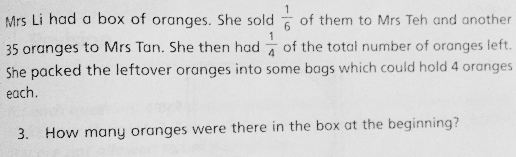QuestionAnyone help me with attached question

Thanks

At first, there were 12u oranges.

1/6 of 12u = 2u

1/4 of 12u = 3u

If she had 1/4 left after selling 2u + 35, then 2u + 35 made up to 3/4 (= 9u) of original.

2u + 35 = 9u

7u = 35

u = 5

In the beginning, there were 12u = 60 oranges.

Yes, there is part 2 question as below:

2. What was the least number of bags she would require to pack the remaining oranges?

Thanks

(a)

number of oranges at first ——- 6u
Mrs Teh ——- 1u
Mrs Tan ——- 35

6u – 1u – 35 = 5u – 35 ——- 6u/4 = 1.5u
5u – 1.5u = 3.5u ——- 35
1u ——- 10
6u ——- 6 x 10 = 60

(b)
60 – 10 – 35 = 15
15/4 = 3R3 = 4 (round up to the nearest whole number)

Ans : (a) 60 oranges; (b) 4 bags.

0 Replies 1 Like

5/6 of the total oranges -35 =1/4 of the total oranges
10/3 of the total oranges – 140= total oranges
7/3 of the total oranges = 140
1/3 of the total oranges = 20
total oranges = 60

0 Replies 1 Like